High Frequency Degrees of Freedom and The Jeans-Landau-Teller Approximation

C. Otey October 31, 2007

(Submitted as coursework for Physics 210, Stanford University, Autumn 2007)Fig.1: Model for a vibrating moleculescattering off an atom.

Introduction

In 1903 Jeans conjectured that high frequency vibrational degrees of freedom in a diatomic gas do not effectively exchange energy with translational degrees of freedom. He argued that in a scattering event this energy transfer should scale exponentially with the frequency of the oscillations.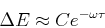for some fixed characteristic energy C and time τ. This approximation was originally intended to explain why vibrational degrees of freedom do not contribute to the specific heat of a diatomic gas at low temperature. The argument was that the energy exchanges involved were so small that the time to reach thermal equilibrium would be large enough to render vibrations ineffective at storing or dissipating thermal energy. Of course quantum mechanics is needed to explain specific heats properly, but Jeans' approximation remains valid and it was revisited by Landau and Teller in the context of the dispersion of sound waves.

This article will demonstrate the ubiquity of the Jeans-Landau-Teller approximation by addressing three systems. All three systems have in common high frequency degrees of freedom interacting with slow degrees of freedom during a scattering event, and all three systems display exponentially small transfer of energy between the fast and slow degrees of freedom.

Vibrating Molecules

Consider the following Hamiltonian representing a vibrating diatomic molecule scattering off an atom-like potential: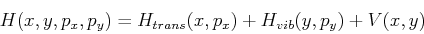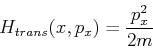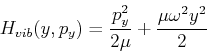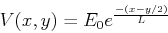Here m is the mass of the molecule, p0 = px(-∞) is the momentum of the incoming molecule, E0 = p02/2m is the initial energy of the molecule, L is a length parameter determining the steepness of the potential, and μ=m/4 is the reduced mass of the oscillator. We assume for simplicity that there are no vibrations at t=-∞, and that the mass of the molecule is evenly divided between the two atoms. We are interested in the behavior of ΔE = Hvib(+∞) as a function of ω. The idea is to assume that the contribution of vibrations to the path of the particle is neglible; that is y(t) << L and Hvib(t) << E0. In this case we can write, setting velocities equal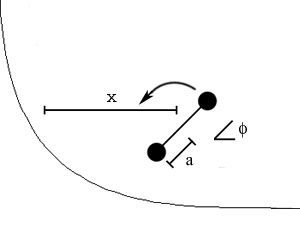Fig. 2: Model for a rotating molecule scattering off an atom.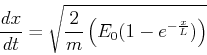If t=0 is the time when x has stopped and turned then x(0) = 0, as this matches the initial kinetic energy of x with the potential at x=0. The solution of the above differential equation for x with this boundary condition is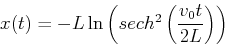which can be verified directly. Now we plug this back into the potential to derive an effective potential for y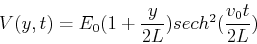which will provide a force for yNow we can calculate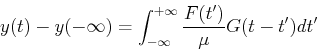where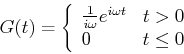is the Green's function of a driven harmonic oscillator. The net transfer of vibrational energy is given by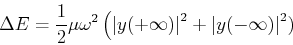In the general case the energy transfer would depend in a complicated way on the initial phase, but we assumed that y(-∞) = 0, so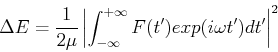We have here the integral of the product of a slowing varying function with a rapidly oscillating function. We expect the result to be small, and when we evaluate the integral we find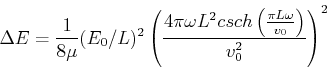If &omega>>v0/L so that there are many vibrations during the collision process, then we have our result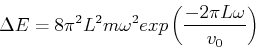where the coefficient 2πL/v0 can be thought of as a characteristic time for the collision process.

Notice that the &omega2 dependence doesn't destroy Jeans' approximation for large ω. But there is a subtlety in the above derivation. In calculating our first order solution for x(t) we ignored y(t) and the change in vibrational energy. For large &omega our result was consistent with our assumption: the energy change is exponentially small at t=∞. However, we have made no guarantees that the vibrational energy change is small near t=0. It turns out this doesn't affect the results, and all of this can be made precise for certain 1D scattering Hamiltonians with arbitrary initial conditions, but this is beyond the scope of this exposition.

Rotating Molecules

Consider another system with a high frequency degree of freedom: a rotating molecule scattering off a steep potential. We can model this with the Hamiltonian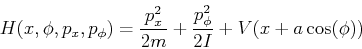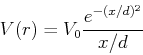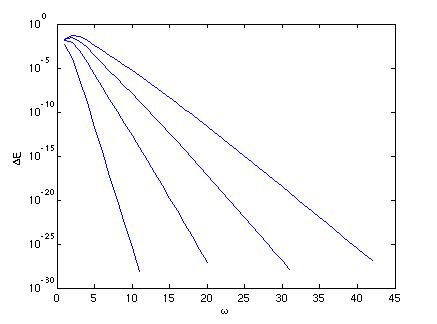Fig. 3: Rotational energy exchange as a function of frequency of rotation for initial Ex=0.25, .5, .75, 1. and I/ma2=100.

The particular form of the potential isn't important, provided it is smooth, steep near x=0 and depends on the rotational configuration in a sensible way. This system is not so amenable to analytical calculations, so we carried out numerical experiments on this system. Excellent agreement to the exponential law was found for large initial rotational frequencies &omega when the results were averaged over the initial phase of the rotation. Care was taken to use a symplectic integrator which guarantees the energy error after the scattering event is O(hnV(x0)) where h is the time step, n is the order of the integrator(4 in this case) and V(x0) is the potential at the point x0 where numerical integration begins and ends[3,4]. The error in energy oscillates substantially near the scattering event(in fact by orders of magnitude greater than the ΔE we are measuring), but then decays to near zero on escape. If a classical integrator like the fourth order Runge-Kutta integrator were used, no similar guarantees on the energy conservation could be made. In practice it was found that ΔE was roughly independent of h below .1*2π/ω and we conclude our calculated ΔE are accurate. The C source code used to calculate the energy changes can be found here.

Gyrating Electrons

Finally, consider two electrons in a strong applied magnetic field. In this case the high frequency degree of freedom is the relative motion of the electrons transverse to the magnetic field as they gyrate about the magnetic field axis at the cyclotron frequency Ω. We will see the exchange of energy between transverse and parallel motions is again exponentially small. The equations of motion can be written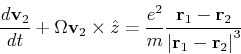By adding and subtracting these equations we get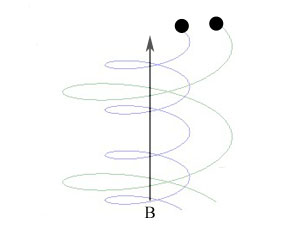Fig. 4: Two electrons gyrating in a magnetic field.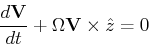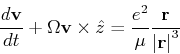where V=d/dt(r1+r2)/2 is the velocity of the center of mass, r=r2-r1 is the position of electron 2 relative to that of electron 1, v=dr/dt is the relative velocity and &mu=m/2 is the reduced mass of the system.

The center of mass motion is just that of an electron in a uniform magnetic field. The relative motion is equivalent to that of an electron in a uniform magnetic field and the field of a fixed charge at r=0. We are interested in the change in transverse kinetic energy, which we again call ΔE. From the equations of motion, the transverse center of mass velocity is conserved, so the change in energy is due entirely to the change in relative velocity, and we can write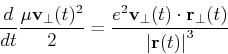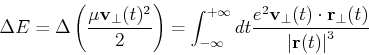As with the vibrational system, we assume that our ΔE is small, so that we can use the first order solution, called the guiding center approximation and rewrite the integral as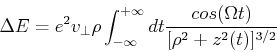where &rho is the guiding center radius and z(t) satisfies a differential equation whose form doesn't matter. The important thing to notice is that again we have the integral of the product of a high frequency oscillation and a slowly varying function, just like in the case of a vibrating molecule scattering off a potential. The integral can be evaluated by deforming the integration into the complex plane, and we get a solution of the form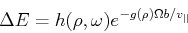where b is the point of closest approach, v|| is the initial relative longitudinal velocity, g(&rho)~&rho/b for large &rho, and h(&omega, &rho) is neither exponentially large nor exponentially small. Once again the Jeans-Landau-Teller approximation holds.

© 2007 C. R. Otey. The author grants permission to copy, distribute and display this work in unaltered form, with attribution to the author, for noncommercial purposes only. All other rights, including commercial rights, are reserved to the author.

References

 J. H. Jeans, Phil Mag. 10:91 (1905).

 L. Landau and E. Teller, Physik. Z. Sowjetunion 11, 18 (1936).

 O. Baldan and G. Benettin, Stat. Phys. 62, 201 (1991).

 J.M. Sanz-Serna and M.P. Calvo, Numerical Hamiltonian Problems, First Edition, (Chapman & Hall, 1994).

 G. Benettin, A. Carati and G. Gallavotti, Nonlinearity 10, 479 (1997).

 D.J. Rapp, Chem. Phys. 32, 735 (1960).

 T.M. O'Neil and P.G. Hjorth, Phys. Fluids 28, 3241 (1985).

 H. Alfvén, Ark. Mat. Astron. Fys. 27A, 1 (1940).